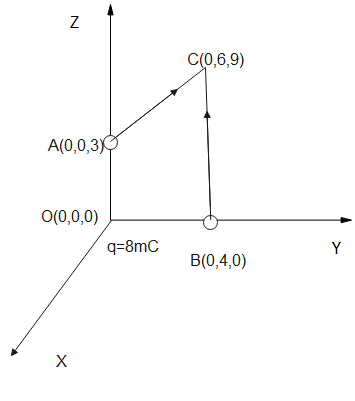Courses
Courses for Kids
Free study material
Offline Centres
MoreLast updated date: 30th Nov 2023
Total views: 279.3k
Views today: 2.79k

# Calculate the work done in taking a charge $-2\times 10^{-9}C$ from A and B via C.Verified
279.3k+ views
Hint: We know that work done is defined as the energy required to move the charge from A to B via C, here, we have two charges, one is given in the question and the other in the diagram, thus we must also consider the effect of force acting between them. Using the formula of work done, we can find the answer.

Complete step-by-step solution:
We know that when two or more charges are kept in the affinity of each other, they exert attractive or repulsive forces depending on the nature of charges present. Here since one is negative and the other is positive, we can say that there is an attractive force which acts between them.
We also know that the change in position of charges or movement of charge from one place to another results in potential difference. Then we can say that the potential energy between the charge is nothing
$V=\dfrac{q}{4\pi\epsilon r}$ where, $q$ is the charge which is kept at a medium whose dielectric constant is $\epsilon$ and the two charges are separated at a distance $r$.
Since the distance of A and B from O is $0.03,\;0.04\;m$ respectively, and the charge at rest is $q_o=8\times 10^{-3}C$ and the moving charge is $-2\times 10^{-9}C$ is say kept at air medium, then the work done is given as
$W=q_o(V_B-V_A)$
$\implies W=\dfrac{q_0q}{4\pi\epsilon}\left(\dfrac{1}{r_b}-\dfrac{1}{r_a}\right)$
$\implies W=9\times 10^{9}\times 8\times 10^{-3}\times-2\times 10^{-9}\times 10^{2}\left(\dfrac{1}{4}-\dfrac{1}{3}\right)$
$\implies W=\dfrac{-144\times10^{-1}}{-12}$
$\therefore W=1.2J$

Note: Since work done due to charges is a path independent function, we must take into account the path followed by charge starting from A to reach B. i.e. we can ignore the point C. Also note that there is a charge $q$ at the origin as shown in the figure.